# Detecting hazy images using hazer

#### 2018-11-01

library(hazer)
library(jpeg)

This vignette exaplains how to 1) perform basic image procssing and 2) estimating image haziness as an indication of fog, cloud or other natural or artificial factors.

Before I start the image processing steps, I show how to read and show and image.

#read the path to the example image
jpeg_file <- system.file(package = 'hazer', 'pointreyes.jpg')

# read the image as an array

# plot the RGB array on the active device panel
par(mar=c(0,0,3,0))
plotRGBArray(rgb_array, bty = 'n', main = 'Point Reyes National Seashore')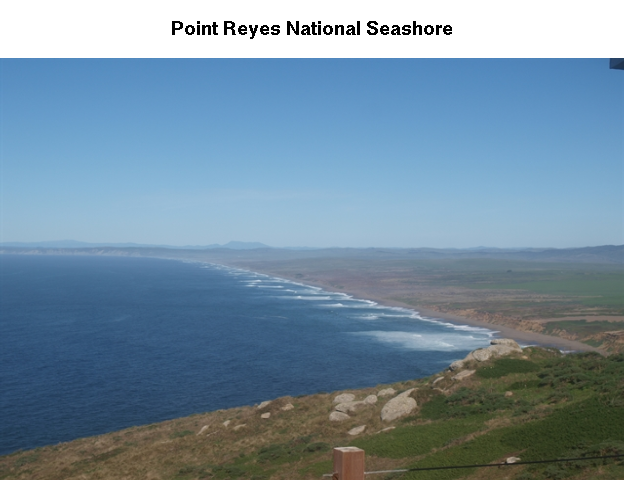#### Histogram of the RGB channels

# color channels can be extracted from the matrix
red_vector <- rgb_array[,,1]
green_vector <- rgb_array[,,2]
blue_vector <- rgb_array[,,3]

# plotting
par(mar=c(5,4,4,2))
plot(density(red_vector), col = 'red', lwd = 2, main = 'Density function of the RGB channels', ylim = c(0,5))
lines(density(green_vector), col = 'green', lwd = 2)
lines(density(blue_vector), col = 'blue', lwd = 2)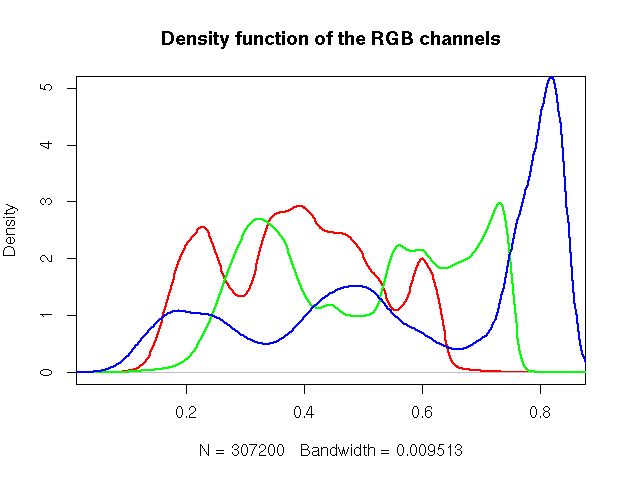## Brightness, Darkness and Contrast

I show how to extract three basic elements of an RGB image using hazer:

1- Extracting brightness of an image 2- Extracting darkness of an image 3- Extracting contrast of an image

#### Brightness of an image

We can extract and show the brightness matrix using the getBrightness function

#extracting the brightness matrix
brightness_mat <- getBrightness(rgb_array)

# unlike the RGB array which has 3 dimensions, the brithness matrix has only two
# dimensions and can be shown as a grayscale image,
# we can do this using the same plotRGBArray function
par(mar=c(0,0,3,0))
plotRGBArray(brightness_mat, bty = 'n', main = 'Brightness matrix')

# each pixel in the brightness image is the maximum of the  R, G and B color channel

# to extract a single brighness value for the image, depending on our needs
#  we can perform some statistics or we can just use the mean of this matrix

# the main quantiles
quantile(brightness_mat)
#>         0%        25%        50%        75%       100%
#> 0.09019608 0.43529412 0.62745098 0.80000000 0.92549020

#  here, we can show the histogram
par(mar=c(5,4,4,2))
hist(brightness_mat)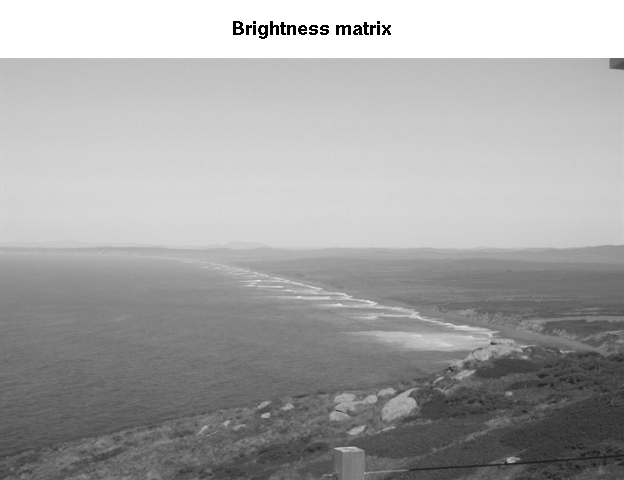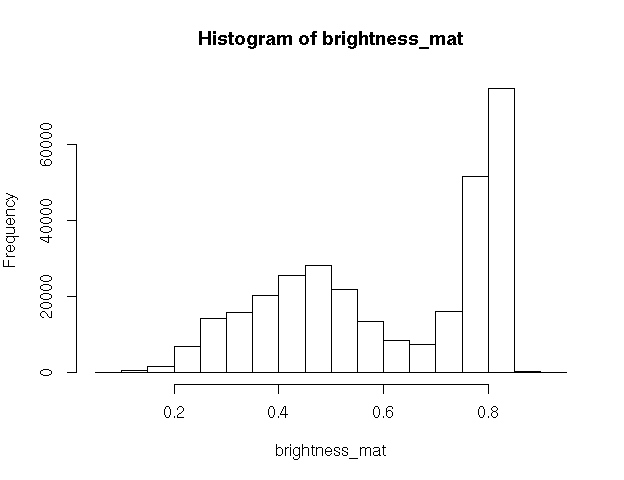#### Darkness of an image

Similarly, we can extract and show the darkness matrix using the getDarkness function

#extracting the darkness matrix
darkness_mat <- getDarkness(rgb_array)

# unlike the RGB array which has 3 dimensions, the darkness matrix has only two
# dimensions and can be shown as a grayscale image,
# we can do this using the same plotRGBArray function

par(mar=c(0,0,3,0))

plotRGBArray(darkness_mat, bty = 'n', main = 'Darkness matrix')

# each pixel in the darkness image is the minimum of the  R, G and B color channel

# similarly, we can do some basic statistics
# the main quantiles
quantile(darkness_mat)
#>         0%        25%        50%        75%       100%
#> 0.03921569 0.23137255 0.36470588 0.47843137 0.81568627

#  here, we can show the histogram
par(mar=c(5,4,4,2))
hist(darkness_mat)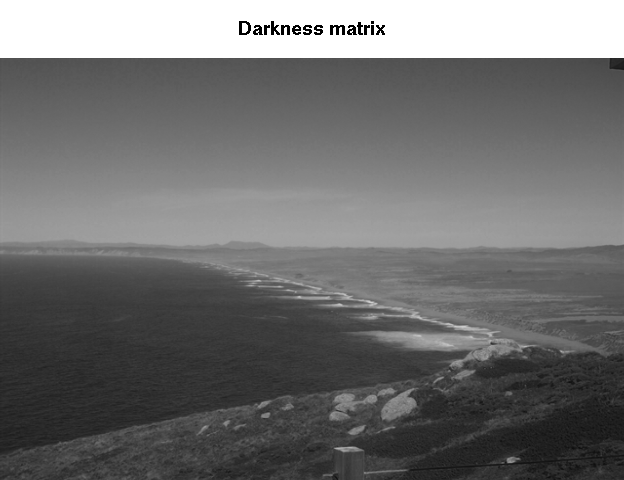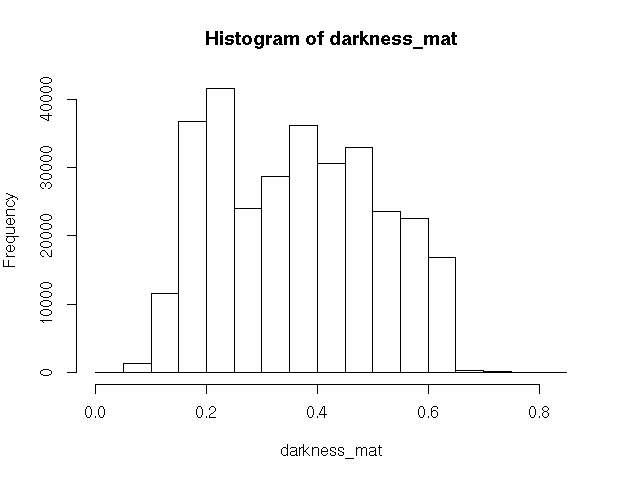#### Contrast of an image

The contrast of the image can quickly be extacted using the getContrast function.

#extracting the contrast matrix
contrast_mat <- getContrast(rgb_array)

# the contrast matrix has also two dimensions and can be shown as a grayscale image
# we can do this using the same plotRGBArray function

par(mar=c(0,0,3,0))

plotRGBArray(contrast_mat, bty = 'n', main = 'Contrast matrix')

# each pixel in the contrast image is the difference between the darkness and brightness matrices

# similarly, we can do some basic statistics
# the main quantiles
quantile(contrast_mat)
#>        0%       25%       50%       75%      100%
#> 0.0000000 0.1450980 0.2470588 0.3294118 0.4392157

#  here, we can show the histogram
par(mar=c(5,4,4,2))
hist(contrast_mat)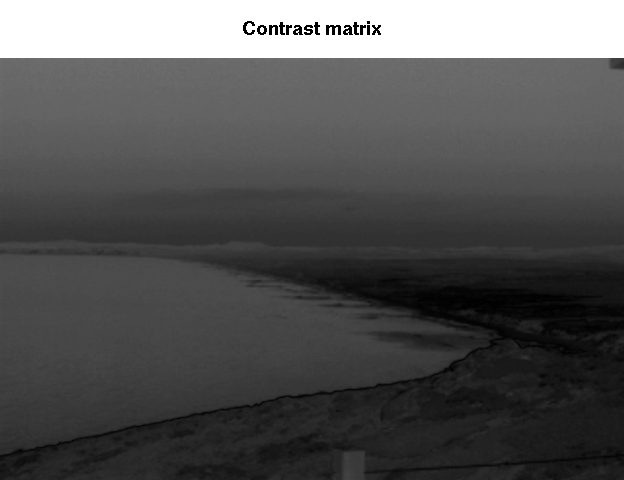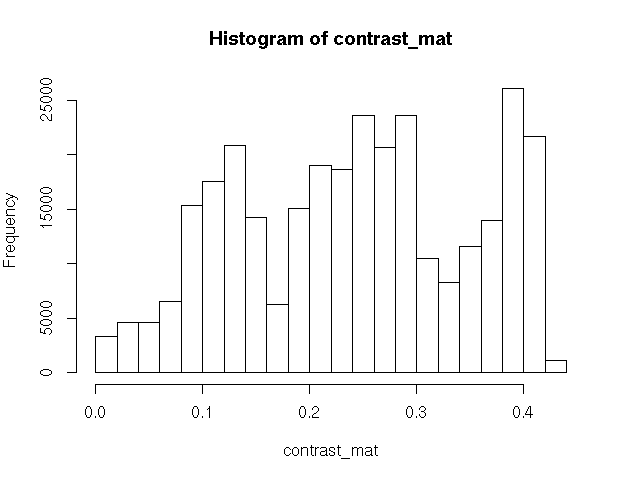## Image fogginess and haziness

Haziness of an image can be estimated using the getHazeFactor function. This function is based on the method described in Mao et al. (2014). The technique was originally developed to for “detecting foggy images and estimating the haze degree factor” for a wide range of outdoor images.

The function returns a list of two numeric values: 1) haze as the haze degree and A0 as the global atmospheric light, as it is explained in the original paper. For the PhenoCam standards, we classify any image with the haze degree greater than 0.4 as a significantly foggy image.

#extracting the haze matrix
haze_degree <- getHazeFactor(rgb_array)

print(haze_degree)
#> $haze #>  0.2247816 #> #>$A0
#>  0.7129889